Warning

# Basic Geometry

## Points and Lines in a Plane

This is a simple task that demonstrates working with homogeneous planar points and lines.

1. Let the image area has an extent [1, 1] to [800, 600]. Draw its boundary.
2. Develop a simple tool, allows to enter two pairs of points within this area and displays them.
3. Calculate the straight line passing through the first pair and the straight line passing through the second pair. Use homogeneous representation. Display the intersection of each line with the image area.
4. Calculate the intersection of both lines and draw it, if it is inside the image area.
5. Apply following homography to all entities and draw the result to another figure.
H = [1     0.1   0;
0.1   1     0;
0.004 0.002 1 ];

Example result of this task is shown in figure 1.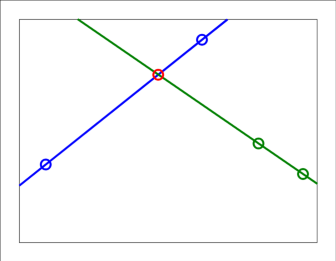Fig. 1: Example intersection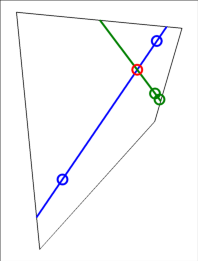Fig. 2: Example intersection under the homography

Notes: the Matlab function ginput is suitable for entering the points.

[u v] = ginput( 4 );
% or
x = ginput(4)';  % note that ginput returns row vectors, hence the transpose

## Perspective camera

Develop a simulation of perspective camera projection – wire-frame model. Let the 3D object be given. The object is composed from two planar diagrams, that are connected. Coordinates of vertices of both diagrams X1 and X2 are:

z = 4;
X1 = [-0.5  0.5 0.5 -0.5 -0.5 -0.3 -0.3 -0.2 -0.2  0  0.5;
-0.5 -0.5 0.5  0.5 -0.5 -0.7 -0.9 -0.9 -0.8 -1 -0.5;
z    z   z    z    z    z    z    z    z  z    z ];
X2 = X1;
X2(3,:) = X1(3,:)+0.5;

Wire-frame model contains edges, that connects vertices in X1 and X2 in given order, and additionally it contains edges connecting vertices between X1 and X2, such that the vertex X1(:,i) is connected to the vertex X2(:,i), ∀ i.

The internal calibration matrix of the camera is:

K = [ 1000    0 500;
0 1000 500;
0    0   1 ];


1. Construct following camera matrices (keep the image u-axis parallel to the scene x-axis):
1. P1: camera in the origin looking in the direction of z-axis.
2. P2: camera located at [0;-1;0] looking in the direction of z-axis.
3. P3: camera located at [0;0.5;0] looking in the direction of z-axis.
4. P4: camera located at [0;-3;0.5], with optical axis rotated by 0.5 rad around x-axis towards y-axis.
5. P5: camera located at [0;-5;4.2] looking in the direction of y-axis.
6. P6: camera located at [-1.5;-3;1.5], with optical axis rotated by 0.5 rad around y-axis towards x-axis (i.e., -0.5 rad) followed by a rotation by 0.8 rad around x-axis towards y-axis. See figure 4.
2. Use the cameras P1 to P6 for projection of given wire-frame model into an image. The edges inside X1 should be drawn red, the edges inside X2 should be drawn blue and the rest should be drawn in black.

The example projection by the camera P1 is shown in figure 3.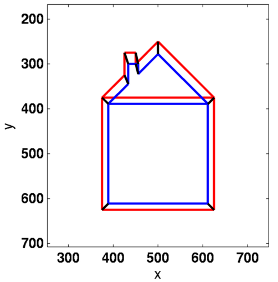Fig. 3: Projection of the wire-frame by the camera P1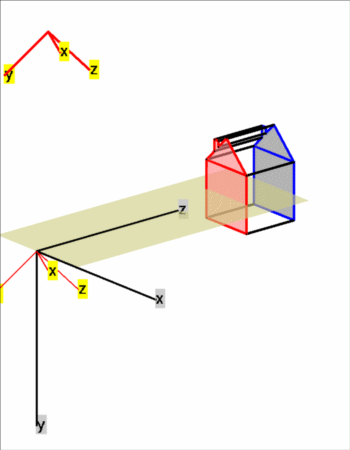Fig. 4: Overview of the P6 situation

Drawing: let u1 = [ u1_1, u1_2, …], v1 be the image coordinates of projected vertices X1 and u2, v2 be the coordinates of projected vertices X2. The desired picture can be drawn, e.g., like this:

plot( u1, v1, 'r-', 'linewidth', 2 )
hold on
plot( u2, v2, 'b-', 'linewidth', 2 )
plot( [u1; u2], [v1; v2], 'k-', 'linewidth', 2 );
set( gca, 'ydir', 'reverse' )
axis equal

## Robust Maximum Likelihood Estimation of a Line(s) From Points

1. Let the image area has an extent [1, 1] to [800, 600].
2. Chose a planar line in general position, suitably inside the image.
3. Randomly generate a set of 800 points belonging to the line. The points are polluted by an isotropic gaussian noise with σ=3 [px]
4. Randomly generate an additional set of 200 outlying points, uniformly distributed over the image area.
5. Mix all the points together.
6. Use the set of all points for (non-robust) ML estimation of the line. Use numeric optimisation (fminsearch)). This is expected to fail.
7. Use the set of all points for robust ML estimation of the line. Use RANSAC to separate inliers and outliersf and than numeric optimisation using the inliers.
8. Plot the whole situation:
• Original line.
• Points.
• Line found by non-robust ML estimations.
• Line found by RANSAC (prior to optimisation)
• Line found by robust ML estimation (RANSAC followed by non-robust optimisation).

## Two Homographies

Consider a pair of images of a scene with two dominant planes, e.g. a pair of images from a triple below. Each plane generate one homography between the image pair. i.e. there are two homographies Ha and Hb.

When a set of tentative correspondences between the images is known (see 2_sparse_correspondences), these homographies can be estimated. Additionally, since these two homographies are generated by two scene planes, there is a line in both images, that is a projection of the intersecting line of the planes. The image points u1, u2 laying on that line in both images are related by both homographies, i.e., u2Ha u1Hb u1, which can be written as inv(Hb) Ha u1 = λ u1. Thus these two homographies must be estimated such that these constraint is enforced (two arbitrary regular 3×3 matrices generally do not ensure the existence of such a common line. Note that this constraint means that there exist a common line that is transformed same way by the two complementary line homographies inv(Ha') and inv(Hb') (this line always exists for any two regular 3×3 matrices) but additionally that this line is transformed by both homographies from the first image to the same line in the second one point-wise.

There are many methods how to estimate multiple geometric models (homographies in this case) from given data. Two simplest possibilities are:

• Sequential RANSAC
1. Estimate the first homography using standard RANSAC scheme for a single homography. Typically the most dominant one is found.
2. Remove inliers of the first found homography from the data.
3. Estimate the second homography using the remaining data, such that the common-line-constraint is enforced (i.e., in each sample, a hypothesis that violates the constraint is not accepted).
• Multi RANSAC with conditional sampling
1. Estimate both homographies simultaneously in RANSAC scheme by sampling 8-tuple of correspondences.
2. In every sample:
1. Draw 4 random correspondences and estimate Ha
2. Remove inliers of Ha from the data set
3. Draw 4 random correspondences and estimate Hb
4. Verify common-line-constraint, reject the hypothesis if violated
5. Verify support of both homographies, accept the best one

Note that having two homographies an inlier must be decided to which it belongs to. This can be done based on minimal error or according to on which side of common line the point is laying.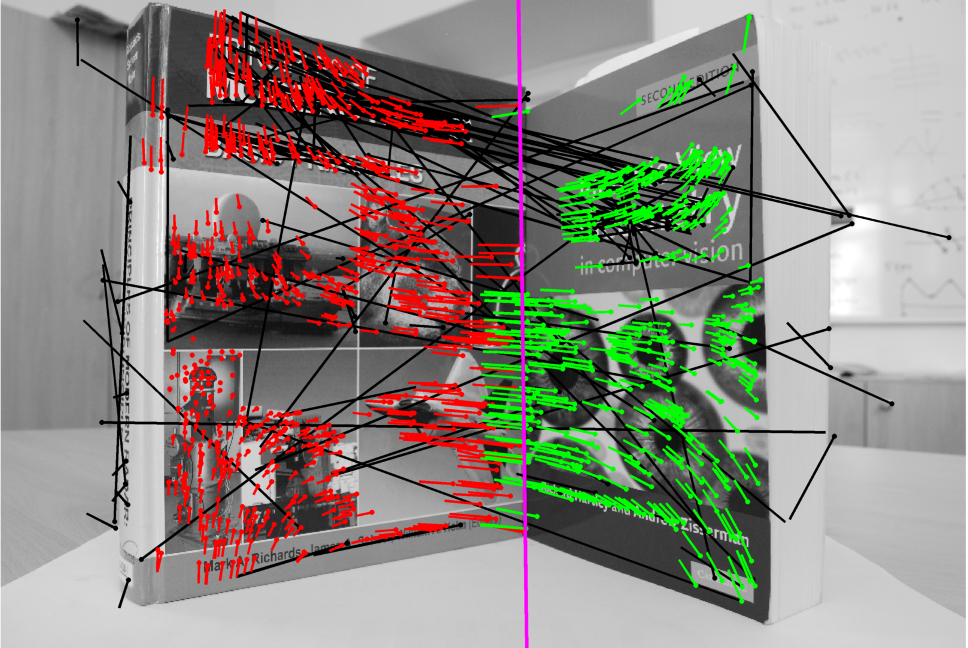Fig. 5: An example of estimated homographies. Red and green =inliers, black = outliers, magenta = common line.